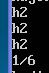﻿ c++ 临时对象的来源_C 语言_脚本之家

# c++ 临时对象的来源

更新时间：2013年01月02日 17:56:54   作者：

#include <iostream>
void swap( int &a,int &b)
{
int temp;
temp=a;
a=b;
b=temp;
}

int main(int argc,char** argv)
{
int a=1,b=2;
swap(a,b);
std::cout<<a<<"-----"<<b<<std::endl;
return 0;
}

结果为
2-----1
可能大多数园友，认为"int temp"是"临时对象"，但是其实不然，"int temp"仅仅是swap函数的局部变量。

#include <iostream>
int countChar(const std::string & s,const char c)
{
int count=0;
for(int i=0;i<s.length( );i++)
{
if(*(s.c_str( )+i) == c)
{
count++;
}
}
return count;
}

int main(int argc,char** argv)
{
char buffer;
char c;
std::cin>>buffer;
std::cout<<"please input the char which you want to chount:";
std::cin>>c;
int count=countChar(buffer,c);
std::count<<"the count is:"<<count<<std::endl;
return 0;
}注意仅当通过传值方式传递对象或者传递常量引用参数，才会发生这类型的转换，当传递非常量引用的参数对象就不会发生。因为传递非常量的引用参数的意图就是想通过函数来改变其传递参数的值，但是函数其实是改变的类型转换建立的临时对象，所以意图无法实现，编译器干脆就直接拒绝。

#include<iostream>
class People
{
public:
People(std::string n,int a)
:name(n),age(a)
{
std::count<<"h2"<<std::endl;
}
People( )
{
std::count<<"h1"<<std::endl;
}
People(const People& P)
{
name=p.name;
age=p.age;
std::cout<<"h3"<<std::endl;
}
std::string name;
int age;
};

void swap(People p1,People p2)
{
People temp;
temp.age=p1.age;
temp.name=p1.name;
p1.age=p2.age;
p1.name=p2.name;
p2.age=temp.age;
p2.name=temp.name;
}

int main(int argc, char ** argv)
{
People p1("tom",18),p2("sam",19);
swap(p1,p2);
return 0;
}void swap(People &p1,People &p2)

#include <iostream>
class Rationanl
{
public:
Rationanl(int e,int d)
:_elemem(e),_denom(d)
{
std::cout<<"h2"<<std::endl;
}
void show( ) const;
int elemem() const {return _elemem;}
int denom() const {return _denom;}
void  setElemon(int e){_elemon=e;}
void  setDenom(int d) {_denom=d;}
Rationanl(const Rationanl &r);
Rationanl & operator=(const Rationanl &r);
private:
int _elemem;
int _denom;
};
Rationanl::Rationanl(const Rationanl &r)
{
setElemon(r.elemon( ));
setDenom(r.denom( ) );
std::cout<<"h3"<<std::endl;
}
Rationanl & Rationanl::operator=(const Rationanl &r)
{
setElemon(r.elemon( ));
setDenom(r.denom( ) );
std::cout<<"h4"<<std::endl;
return *this;
}

void Rationanl::show( )
{
std::cout<<_elemen<<"/"<<_denom<<std::endl;
}
const Rationanl operator*(const Rationanl lhs,const Rationanl rhs)
{
return Rational result(lhs.elemen*rhs.elemen,rhs.denom*rhs.denom);
}

int main(int argc,char **argv)
{
Rationanl r1(1,2),r2(1,3)
Rationanl r3=r1*r2;    //GCC做了优化，没有看到临时变量。编译器直接跳过建立r3,使用赋值符号
r3.show( );
//相当于  (r1*r2).show( );
return 0;
}